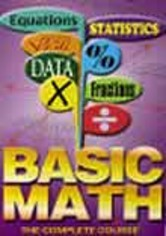# Basic Math: Lesson 30: Introduction to Algebra I

1998 NR 0h 30m DVD# Basic Math: Lesson 30: Introduction to Algebra I

1998 NR 0h 30m DVD
• Overview
• Details
The final installment of the Basic Math series introduces the elements of algebra, explaining its relationship to the arithmetic learned earlier in lessons 1-29 and providing a comprehensive review of the cumulative material. By the end of the lesson, you will have learned the rules of adding, subtracting and multiplying fractions; the definition of a polynomial; and the arithmetic of polynomials as compared to the arithmetic of whole numbers.
Cast
Murray Siegel
Format
DVD
Screen
Full Screen 1.33:1
CC
No
Audio
English: Dolby Digital 2.0 Stereo
Rating
NR - Not rated. This movie has not been rated by the MPAA.

## How it works

• 1

Browse from thousands of movies and shows.

• 2

#### Free delivery